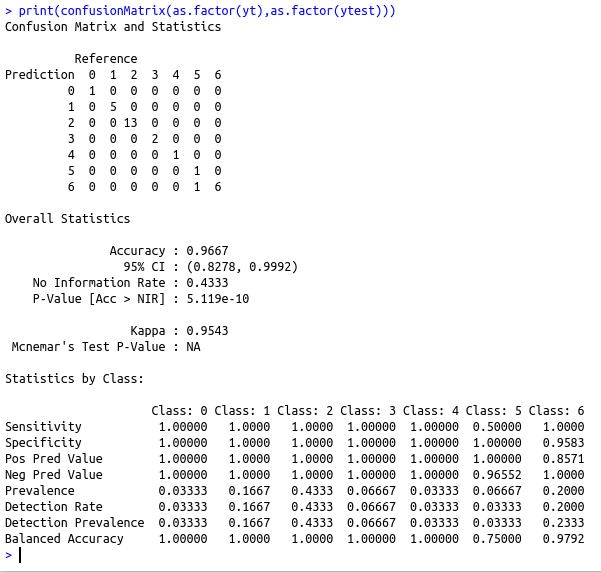• #5, First Floor, 4th Street Dr. Subbarayan Nagar Kodambakkam, Chennai-600 024 Landmark : Samiyar Madam
• pro@slogix.in
• +91- 81240 01111

### How to classify animals into different groups by their characteristics using deep neural networks in R?

###### Description

To classify animals into different groups by their characteristics using deep neural networks in R

#### Functions Used :

model
layer_dense() – Add a input,output or hidden layer
layer_activation() – Activation function for the layer
compile() – To compile the model
fit() – To fit the model using the train set
predict() – To predict using the test set
evaluate() – To evaluate the loss and metrics

#### Libraries required :

library(keras)

###### Process

Convert the categorical variables to equivalent numeric classes

Split the data set as train set and test set

Initialize the keras sequential model

Build the model with input layers,hidden layers and output layer as per the data size along with the activation function(here 16 I/P layer with relu activation and 7 O/P layer with softmax activation)

Compile the model with required loss,metrics and optimizer(here loss=categorical_crossentropy,optimizer=adam,metrics=accuracy)

Fit the model using the train set

Predict using the test set

Evaluate the metrics

###### Sapmle Code

library(caret)
library(keras)
#Covert the categorical data into numeric class
data\$Type=factor(data\$Type,labels=c(0:6))
#To Split 70% of data as training data
smp_size train_ind #Train set
train_data<-data[train_ind,]
xtrain=train_data[,2:17]
ytrain=train_data\$Type
#Test set
test=data[-train_ind,]
xtest=test[,2:17]
ytest=test\$Type
#converting the target variable to once hot encoded vectors using keras inbuilt function
train_y<-to_categorical(ytrain)
test_y #defining a keras sequential model
model %
layer_dense(units = 30, input_shape = 16 )%>%
layer_activation(activation = ‘relu’) %>%
layer_dense(units = 7)%>%
layer_activation(activation= “softmax”)
#compiling the defined model with metric = accuracy and optimiser as adam.
model %>% compile(
loss = ‘categorical_crossentropy’,
metrics = ‘accuracy’
)
#Summary of the model
summary(model)
#fitting the model on the training dataset
model %>% fit(as.matrix(xtrain), train_y, epochs = 100,batch_size=10)
#Predict using the Test data
yt=predict_classes(model,as.matrix(xtest))
loss_and_metrics % evaluate(as.matrix(xtest), test_y)
print(loss_and_metrics)
cat(“The confusion matrix is \n”)
print(confusionMatrix(as.factor(yt),as.factor(ytest)))

###### Screenshots